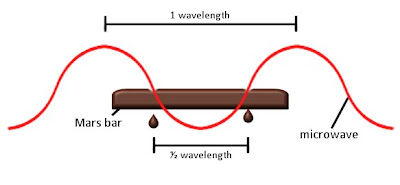## Wednesday, February 17, 2010

### Chocolate physics experimentA left over chocolate bar from Valentine's Day may be used to measure the speed of light.

"Mid Morning Experiment: Speed of Light"

by

Mark Steer

Null Hypothesis: TheJournal of Unlikely Science

Einstein realised that the speed of light was one of the defining measures of the Universe. Remember E=mc2, the beautiful little formula that wrapped up the theory of relativity? Well ‘c’ is the speed of light. And you can measure it. All you need is a microwave, a ruler and a bar of chocolate (and maybe a calculator).

What to do

1.) Remove the revolving tray from your microwave.

2.) On a plate, heat a chocolate bar on full power until it starts to melt in two or three places – this will probably only take about 20 seconds.

3.) Remove the chocolate from the microwave and measure the distance between the melted spots.

4.) Assume your microwave is a standard one which has a frequency of 2.45 gigahertz (you could check this in the back of the manual if you know where it is).

5.) Multiply the distance between the spots on the chocolate bar by 2 and then by 2,450,000,000 (which 2.45 gigahertz expressed as hertz).

6.) Fall off your chair as your result is astoundingly close to 299,792,458, metres per second - the speed of light. (Remember that if you’ve measured the distance in cm then you’ll have to divide your answer by 100).

What happensMeasuring the distance between the two melted spots means we can work out the wavelength of the microwaves. And if we know the wavelength and the wave frequency, we can work out the speed at which the waves are travelling.

Working out the wavelength

Because the chocolate bar stays still in the microwave, the waves keep hitting it in the same places - the bits which get hot and melt. The distance between the melted spots is the distance of half a wavelength since the microwave passes through the bar both on its way up and way down. Multiplying the distance by two gives us the entire length of one wave.

Why the frequency is important

Microwaves, like light waves, are a form of electromagnetic radiation which travel at light speed. In your oven they have a frequency of 2.45 gigahertz, which means that they bounce up and down 2.45 billion times every second. We have already worked out the wavelength - distance a wave travels when it goes through one up and down cycle - therefore we can work out how far the wave would go in 2.45 billion cycles or, in other words, how far it would go in a second.

Calculating the speed of light

If you found that the distance between the spots was 6cm then 6 x 2 x 2,450,000,000 (2.45 billion) would give you a result of 29,400,000,000 cm per second, or 294,000,000 metres per second – a result which is blinking similar to the real speed of light that physicists spent the best part of half a century trying to measure.Incidentally, the cooking power of microwaves was first discovered when a scientist stood too close to a microwave experiment with a chocolate bar in his pocket. It melted, the scientist was intrigued and the microwave oven was born.